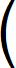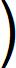Home  │ Audio Home Page

Created November 21, 2014.  See Document History at end for details.

# Decibels

The calculation and use of decibels in audio

### Introduction

Because human senses – including hearing – perceive signal levels exponentially (or logarithmically depending on point of view), it was deemed useful to represent the levels of these signals by a logarithm of their magnitude.  As a result the Bell(B) – named after Alexander Graham Bell – was designated to be the unit representing the base ten logarithm of signal power ratio.

 (1) powerBell = log10(power-ratio)

These units are rather large for many uses so the smaller unit of decibel (dB) became more commonly used.  In this system, level differences of 1dB are just noticeable, 3dB more so, and 10dB is a significant change.

 (2) powerdB = 10 × log10(power-ratio)

Because power is proportional to the square of voltage (or current) decibels can represent voltage or current ratios as well.

 (3) voltagedB = 20 × log10(voltage-ratio)

Used alone dB can represent voltage or power ratios and which is inferred must be determined by context.  Additionally, dB also is used to represent absolute values against some fixed reference.  Examples used in electronics and audio are

Figure 1: Absolute decibel units

 Unit Quantity represented Reference value Calculation dBV Voltage 1V 20 × log10(voltage) dBW Power 1W 10 × log10(power) dB(SPL) Sound pressure level 20µPa 20 × log10(sound-pressure / 2×10–5Pa ) dB(SWL) Sound power or intensity 1pW (10–12W) 10 × log10(sound-power / 10–12W)

Reference levels for dB(SPL) and dB(SWL) were chosen so that they produced the same decibel values for the same sound level.  The following table relates decibel sound levels to their measurements and to some common sound experiences.

Figure 2:  Sound pressure level related data1

 Decibels (dB(SPL) or dB(SWL)) Sound pressure level (Pa or N/m2) Intensity (W/m2) Type of sound 130 63.2456 10 artillery fire at close proximity (threshold of pain) 120 20 1 amplified rock music; near jet engine 110 6.32456 10–1 loud orchestral music, in audience 100 2 10–2 electric saw 90 6.32456×10–1 10–3 bus or truck interior 80 2×10–1 10–4 automobile interior 70 6.32456×10–2 10–5 average street noise; loud telephone bell 60 2×10–2 10–6 normal conversation; business office 50 6.32456×10–3 10–7 restaurant; private office 40 2×10–3 10–8 quiet room in home 30 6.32456×10–4 10–9 quiet lecture hall; bedroom 20 2×10–4 10–10 radio, television, or recording studio 10 6.32456×10–5 10–11 soundproof room 0 2×10–5 10–12 absolute silence (threshold of hearing)

### Relating speaker sensitivity and power to resulting sound level

Calculations relating speaker sensitivity, power,  and sound level seem the most useful for audio hobbyists.  Speaker sensitivity often has to be recalculated because it is not power sensitivity as thought.  Because sensitivity specifications are given specifically for 8Ω it instead represents voltage sensitivity referred to the voltage necessary to drive 1W into 8Ω, that is 2.82843V.  Before power decibels can be added to speaker sensitivity to give sound level, sensitivity has to be in power decibels.

To convert speaker sensitivity to power decibels.
 (4) sensitivitypower = sensitivityvoltage + dBspeaker impedance 8ΩThen sound level can be calculated.
 (5) volumedB(SPL) = sensitivitypower + powerdBW

Example:
A speaker of 90dB/1W sensitivity will produce a sound level of 117dBSPL when driven with 17dB (50W) of power.

Scientific calculators are not always handy when you want to do decibel calculations.  However because decibels are logarithms, the laws of logarithms simplify calculations.

Figure 3: Some basic laws of logarithms in decibel form

 dB(x × y) = dB(x) + dB(y) dB(x / y) = dB(x) – dB(y) dB(1 / x) = –dB(x) dB(xy) = dB(x) × y

From a few known decibel relations, the remainder can be calculated in your head or on paper without undue difficultly, so long as calculations are short enough not to accumulate error approaching 1dB.

Figure 4: A table of decibels and now they would have been derived from head math

Ratio

dBW
dBV
Calculating decibels from estimated ratio (you can infer how to calculate in the other direction)

1

0
0
A given
1.25893

1
2
dB(1.25) = dB(10/23) = dB(10) – (dB(2) × 3) = 10dB – (3dB × 3) = 1dB
1.58489

2
4
dB(3.16228 / 2) = dB(3.16228) – dB(2) = 5dB – 3dB = 2dB
1.99526

3
6
Round to 2.  This is a given you would expect to know and begin with.
2.51189

4
8
dB(2.5) = dB(10/(2 × 2)) = dB(10) – dB(2) – dB(2) = 10dB – 3dB – 3dB = 4dB
3.16228

5
10
 dB( 10 ) = dB(10) / 2 = 10dB/2 = 5dB (It is helpful to remember this one too.)
3.98107

6
12
dB(4) = dB(2 × 2) =  dB(2) + dB(2) = 3dB + 3dB = 6dB
5.01187

7
14
dB(5) = dB(10 / 2) =  dB(10) – dB(2) = 10dB – 3dB = 7dB
6.30957

8
16
dB(3.16228 × 2) = dB(3.16228) + dB(2) = 5dB + 3dB = 8dB
7.94328

9
18
dB(23) = dB(2) × 3 = 3dB × 3 = 9dB
10

10
20
A given
100

20
40
A given
1000

30
60
A given

### Example Calculations

Example 1:  Determine the sound pressure level to which a 30W amp will drive a 6Ω speaker of 90dB sensitivity.

From equation 4 calculate speaker sensitivity.
 (6) sensitivitypower = 90dB + dB6Ω 8Ω= 90dB + dB3 4= 90dB + dB3 2 × 2(7) sensitivitypower ≈ 90dB + dB(3.16228) – (dB(2) + dB(2)) = 90dB + 5dB – (3dB + 3dB)

 (8) sensitivitypower ≈ 89dB/W (88.7506dB by calculator)

Calculate power using head math and table of decibels in figure 4.
 (9) powerdBW = dB(30W) = dB(10W × 3) = dB(10) + dB(3.16228) = 10dB + 5dB

 (10) powerdBW = 15dB

Finally calculate sound pressure level using equation 5.
 (11) volumedB(SPL) = 89dB + 15dBW = 104dB(SPL)

Example 2:  Determine power required to drive a 4Ω speaker of 88dB sensitivity to 110dB.

From equation 4 calculate speaker sensitivity.
 (12) sensitivitypower = 88dB + dB4Ω 8Ω= 88dB + dB1 2(13) sensitivitypower = 88dB – dB(2) = 88dB – 3dB = 85dB/W

Calculate power required by reversing equation 5.
 (14) powerdBW  = volumedB(SPL) – sensitivitypower = 110dB – 85dB = 25dBW

Convert power in watts to decibels.
 (15) power  =  dB-1(25dBW) = dB-1(20dBW + 5dBW) = dB-1(20dBW) × dB-1(5dBW)

 (16) power  =  (100 x 3.16)W = 316W

1Table partially based on Encyclopedia Britannica, "Table 1: Sound Levels for Nonlinear (Decibel) and Linear (Intensity) Scales," 2002.

Document History
November 21, 2014  Created.